# Texas Go Math Grade 6 Module 3 Answer Key Multiplying and Dividing Fractions

Refer to our Texas Go Math Grade 6 Answer Key Pdf to score good marks in the exams. Test yourself by practicing the problems from Texas Go Math Grade 6 Module 3 Answer Key Multiplying and Dividing Fractions.

## Texas Go Math Grade 6 Module 3 Answer Key Multiplying and Dividing Fractions

Write each improper fraction as a mixed number.

Question 1.
$$\frac{9}{4}$$ ____________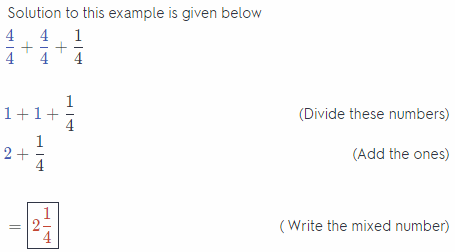Question 2.
$$\frac{8}{3}$$ ____________Question 3.
$$\frac{23}{6}$$ ____________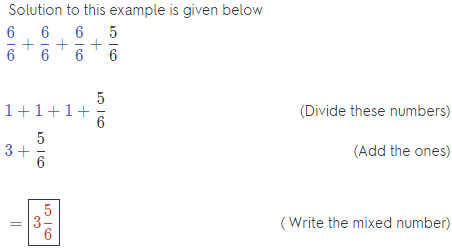$$\frac{11}{2}$$ ____________Question 5.
$$\frac{17}{5}$$ ____________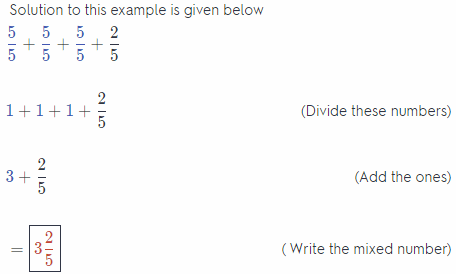Question 6.
$$\frac{15}{8}$$ ____________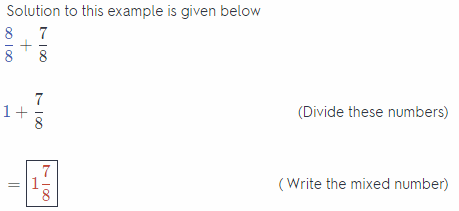Question 7.
$$\frac{33}{10}$$ ____________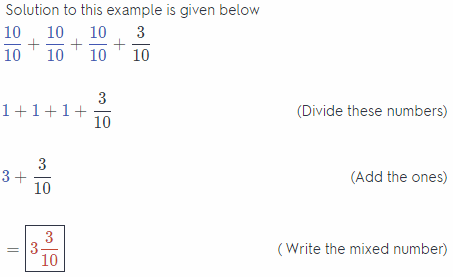Question 8.
$$\frac{29}{12}$$ ____________Multiply

Question 9.
6 × 5 ____________
Use related fact you know
5 × 5 = 25

Think: 6 × 5 = (5 × 5) + 5
= 25 + 5
= 30

Question 10.
8 × 9 ____________
Use related fact you know
8 × 8 = 64

Think: 8 × 9 = (8 × 8) + 8
= 64 + 8
= 72

Question 11.
10 × 11 ____________
Use related fact you know
10 × 10 = 100

Think: 10 × 11 = (10 × 10) + 10
= 100 + 10
= 110

Question 12.
7 × 8 ____________
Use related fact you know
7 × 7 = 49

Think: 7 × 8 = (7 × 7) = 7
= 49 + 7
= 56

Question 13.
9 × 7 ____________
Use related fact you know
7 × 7 = 49

Think: 9 × 7 = (7 × 7) + 7 + 7
= 49 + 14
= 63

Question 14.
8 × 6 ____________
Use related fact you know
6 × 6 = 36

Think: 8 × 6 = (6 × 6) + 6 + 6
= 36 + 12
= 48

Question 15.
9 × 11 ____________
Use related fact you know
9 × 9 = 81

Think 9 × 11 = (9 × 9) + 9 + 9
= 81 + 18
= 99

Question 16.
11 × 12 ____________
Use related fact you know
11 × 11 = 121

Think 11 × 12 = (11 × 11) + 11
= 121 + 11
= 132

Divide.

Question 17.
35 ÷ 7 ____________
Solution to this example is given below

Think: 7 times what number equal 35?
7 × 5 = 35
so, 35 ÷ 7 = 5

Question 18.
56 ÷ 8 ____________
Solution to this example is given below

Think: 8 times what number equal 56?
8 × 7 = 56
so, 56 ÷ 8 = 7

Question 19.
28 ÷ 7 ____________
Solution to this example is given below

Think: 7 times what number equal 28?
7 × 4 = 28
so, 28 ÷ 7 = 4

Question 20.
48 ÷ 8 ____________
Solution to this example is given below

Think: 8 times what number equal 48?
8 × 6 = 48
so, 48 ÷ 8 = 6

Question 21.
36 ÷ 4 ____________
Solution to this example is given below

Think: 4 times what number equal 36?
4 × 9 = 36
so, 36 ÷ 4 = 9

Question 22.
45 ÷ 9 ____________
Solution to this example is given below

Think: 9 times what number equal 45?
9 × 5 = 45
so, 45 ÷ 9 = 5

Question 23.
72 ÷ 8 ____________
Solution to this example is given below

Think: 8 times what number equal 72?
8 × 9 = 72
so, 72 ÷ 8 = 9

Question 24.
40 ÷ 5 ____________
Solution to this example is given below

Think: 5 times what number equal 40?
5 × 8 = 40
so, 40 ÷ 5 = 8

Visualize Vocabulary
Use the ✓ words to complete the triangle. Write the review word that fits the description in each section of the triangle.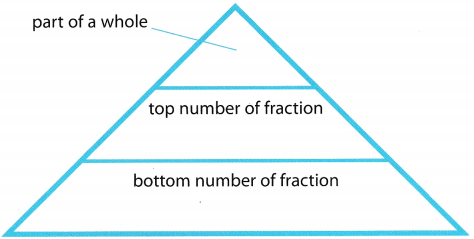Understand Vocabulary

In each grouping, select the choice that is described by the given vocabulary word.

Question 1.
reciprocals
(A) 1:15
(B) $$\frac{3}{4} \div \frac{1}{6}$$
(C) $$\frac{3}{5}$$ and $$\frac{5}{3}$$
If the product of two numbers is 1, then the two numbers are said to be reciprocals of each other.

Only viable Solution is C, so let’s check it!
$$\frac{3}{5} \times \frac{5}{3}=\frac{15}{15}$$ = 1
Therefore, this solution is valid1

Question 2.
Mixed Number
(A) $$\frac{1}{3}-\frac{1}{5}$$
(B) 3$$\frac{1}{2}$$
(C) – 5
Mixed number is a number that is a combination of a whole number and a fraction.

Only B answer is Combination of whole number and a fraction!

Question 3.
Whole Number
(A) – 1
(B) 7
(C) $$\frac{2}{5}$$